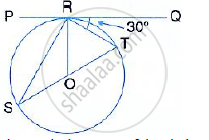Share

If Pq is a Tangent to the Circle at R; Calculate: (I) ∠Prs (Ii) ∠Rot Given O is the Centre of the Circle and Angle Trq = 30° - ICSE Class 10 - Mathematics

ConceptTangent Properties - If a Line Touches a Circle and from the Point of Contact, a Chord is Drawn, the Angles Between the Tangent and the Chord Are Respectively Equal to the Angles in the Corresponding Alternate Segments

Question

If PQ is a tangent to the circle at R; calculate:
(i) ∠PRS (ii) ∠ROTGiven O is the centre of the circle and angle TRQ = 30°

Solution

PQ is a tangent and OR is the radius.
∴ OR ⊥  PQ
∴ ∠ORT = 90°

⇒ ∠TRQ = 90° - 30° = 60°
But in Δ OTR ,
OT = OR (Radii of the same circle)

∴ ∠OTR = 60° Or ∠STR = 60°
But,
∠PRS = ∠STR = 60 (Angle in the alternate segment)
In ΔORT,
∠ORT  = 60°
∠OTR =60°
∴ ∠ROT = 180° -(60° +60° )
∴ ∠ROT = 180°  - 120° = 60°

Is there an error in this question or solution?

Video TutorialsVIEW ALL 

Solution If Pq is a Tangent to the Circle at R; Calculate: (I) ∠Prs (Ii) ∠Rot Given O is the Centre of the Circle and Angle Trq = 30° Concept: Tangent Properties - If a Line Touches a Circle and from the Point of Contact, a Chord is Drawn, the Angles Between the Tangent and the Chord Are Respectively Equal to the Angles in the Corresponding Alternate Segments.
S